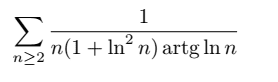# Convergence of a series involving ln() terms in the denominator of a fraction

Amaelle
Homework Statement:
the convergence of a series (look at the image)
Relevant Equations:
asymptotic comparison
good day
I want to study the convergence of this serie and want to check my approchI want to procede by asymptotic comparison
artgln n ≈pi/2
n+n ln^2 n ≈n ln^2 n
and we know that
1/(n ln^2 n ) converge so the initial serie converge

#### Attachments

Mentor
2022 Award
This approach is far too hand wavy. You have no control about the approximations, and they even occur in the denominator! If you want to use the majority criterion you need an estimation
$$\dfrac{1}{n(1+\log^2(n))\tan^{-1}(\log(n))}\leq \dfrac{1}{n^c} \Longleftrightarrow n^c\leq n(1+\log^2(n))\tan^{-1}(\log(n))$$
for some ##c > 1##. This means you have to find a lower bound for the denominator which is still big enough.

••docnet and Amaelle
Homework Helper
Gold Member
I want to procede by asymptotic comparison
artgln n ≈pi/2
n+n ln^2 n ≈n ln^2 n
You should make this stronger. n+n ln^2 n > n ln^2 n > 0, so |1/(n + n ln^2 n )| < |1/(n ln^2 n )|.
Amaelle said:
and we know that
1/(n ln^2 n ) converge so the initial serie converge
Do you know that it is absolutely convergent? If so, say that.

Last edited by a moderator:
••docnet and Amaelle
Amaelle
thank you so much so my approach , (if I write it as you did ) is correct? I can use the approximation lim artgln n ≈pi/2 when n tends to infinity?

Amaelle
This approach is far too hand wavy. You have no control about the approximations, and they even occur in the denominator! If you want to use the majority criterion you need an estimation
$$\dfrac{1}{n(1+\log^2(n))\tan^{-1}(\log(n))}\leq \dfrac{1}{n^c} \Longleftrightarrow n^c\leq n(1+\log^2(n))\tan^{-1}(\log(n))$$
for some ##c > 1##. This means you have to find a lower bound for the denominator which is still big enough.
thank you very much , I got your point!

Staff Emeritus
Gold Member
What is artg?

Amaelle
the function arctangent

Staff Emeritus
••